﻿ 简单明朗的 RNN 写诗教程_其他_开心洋葱网
• 欢迎访问开心洋葱网站，在线教程，推荐使用最新版火狐浏览器和Chrome浏览器访问本网站，欢迎加入开心洋葱` QQ群`
• 为方便开心洋葱网用户，开心洋葱官网已经开启复制功能！
• 欢迎访问开心洋葱网站，手机也能访问哦~欢迎加入开心洋葱多维思维学习平台` QQ群`
• 如果您觉得本站非常有看点，那么赶紧使用Ctrl+D 收藏开心洋葱吧~~~~~~~~~~~~~！
• 由于近期流量激增，小站的ECS没能经的起亲们的访问，本站依然没有盈利，如果各位看如果觉着文字不错，还请看官给小站打个赏~~~~~~~~~~~~~！

# 简单明朗的 RNN 写诗教程

1152次浏览

• 简单明朗的 RNN 写诗教程
• 数据集介绍
• 代码思路
• 输入 and 输出
• 训练集构建
• 生成一首完整的诗
• 代码实现
• 读取文件
• 统计字数
• 构建word 与 id的映射
• 转成one-hot代码
• 随机打乱数据
• 构建训练集
• 构建模型
• 批加载数据
• 训练的过程中生成诗句
• 开始训练
• 诗词生成
• 总结
• 参考

# 简单明朗的 RNN 写诗教程## 数据集介绍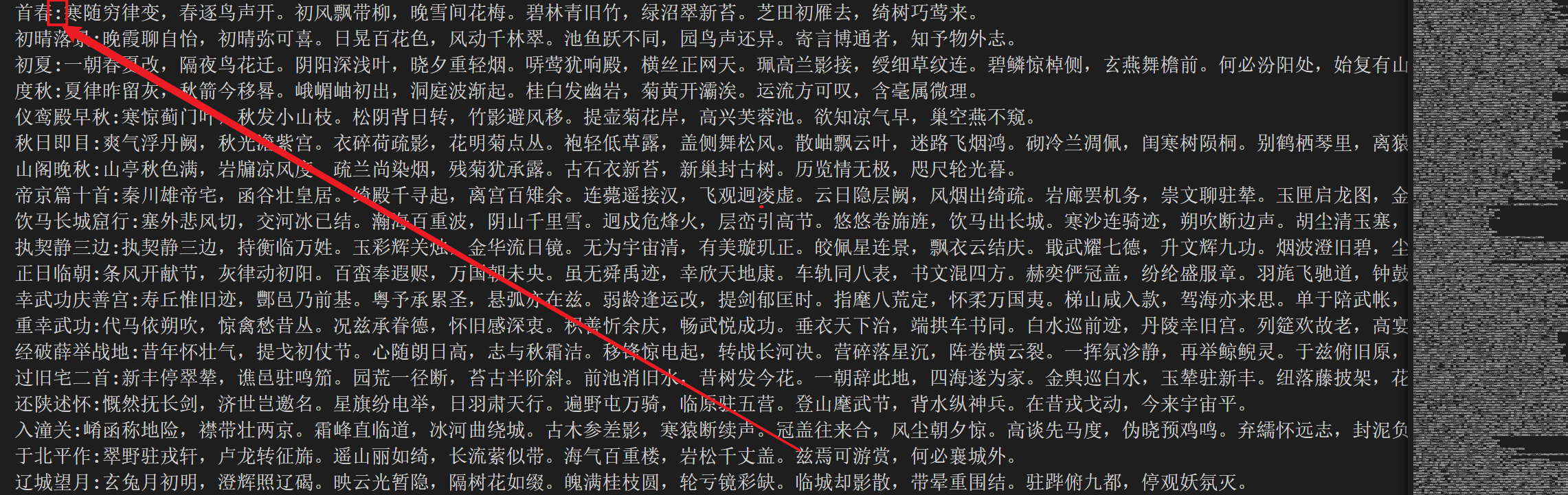## 代码思路

### 输入 and 输出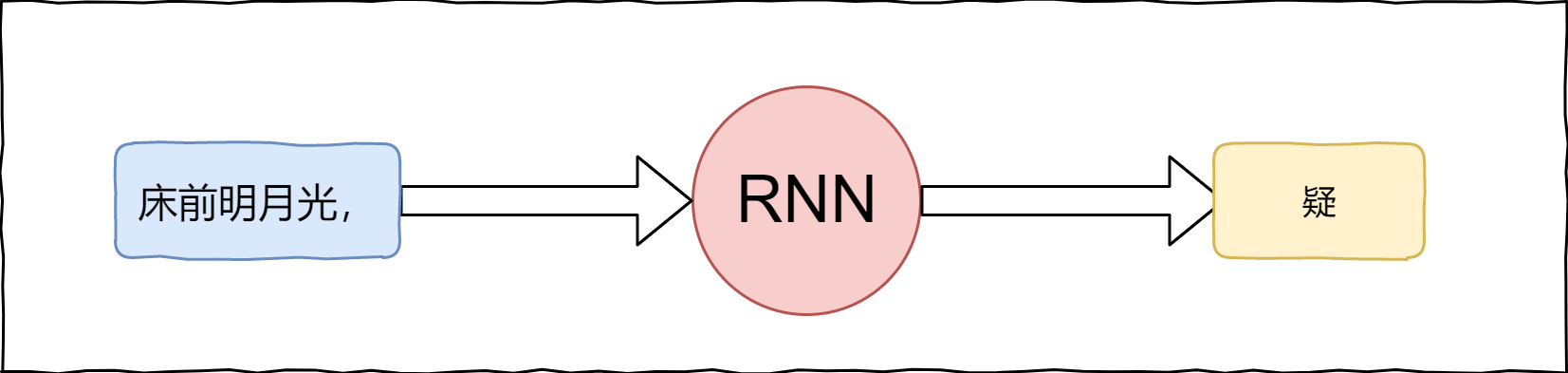RNN接受 6个字符（5个字+一个标点符号），然后输出下一个字符。至于怎么生成一首完整的诗词，等到后面讨论。

RNN当然不能够直接接受 “床前明月光，” 这个中文的输入，我们要对其进行 Encode，变成数字，然后才能够输入到RNN网络中。同理，RNN输出的肯定也不是一个中文字符，我们也要对其进行Decode 才能将输出变成一个中文字符。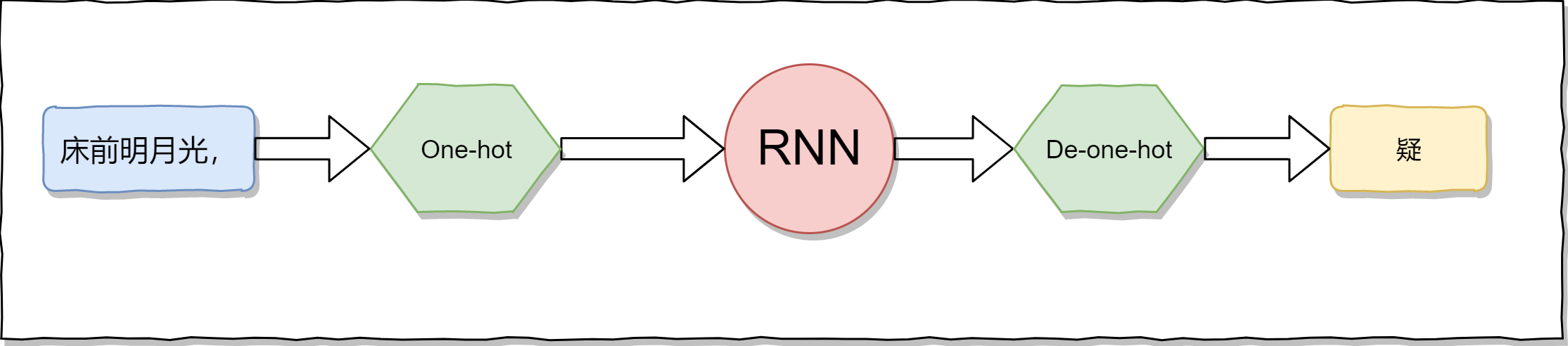### 训练集构建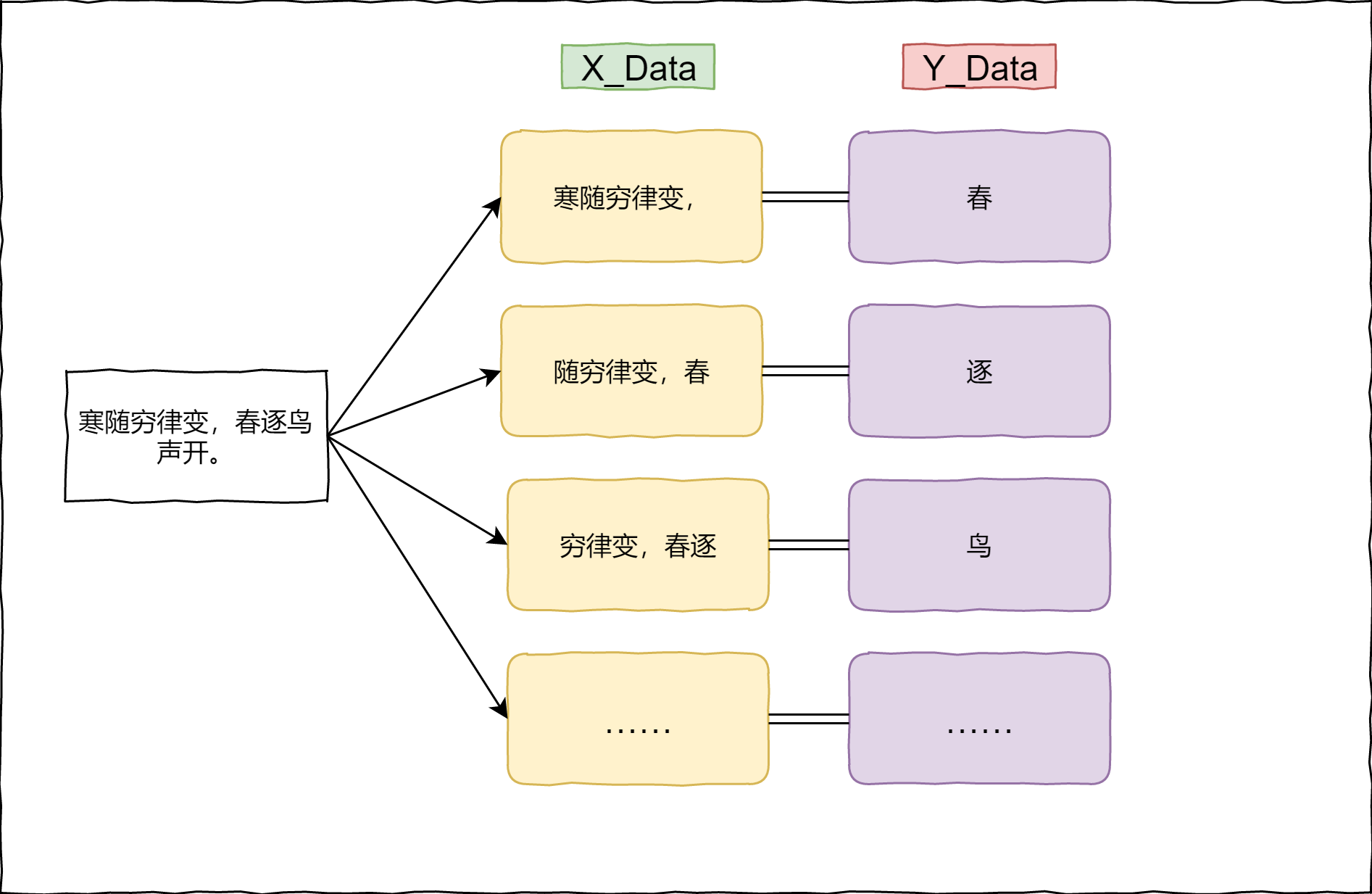### 生成一首完整的诗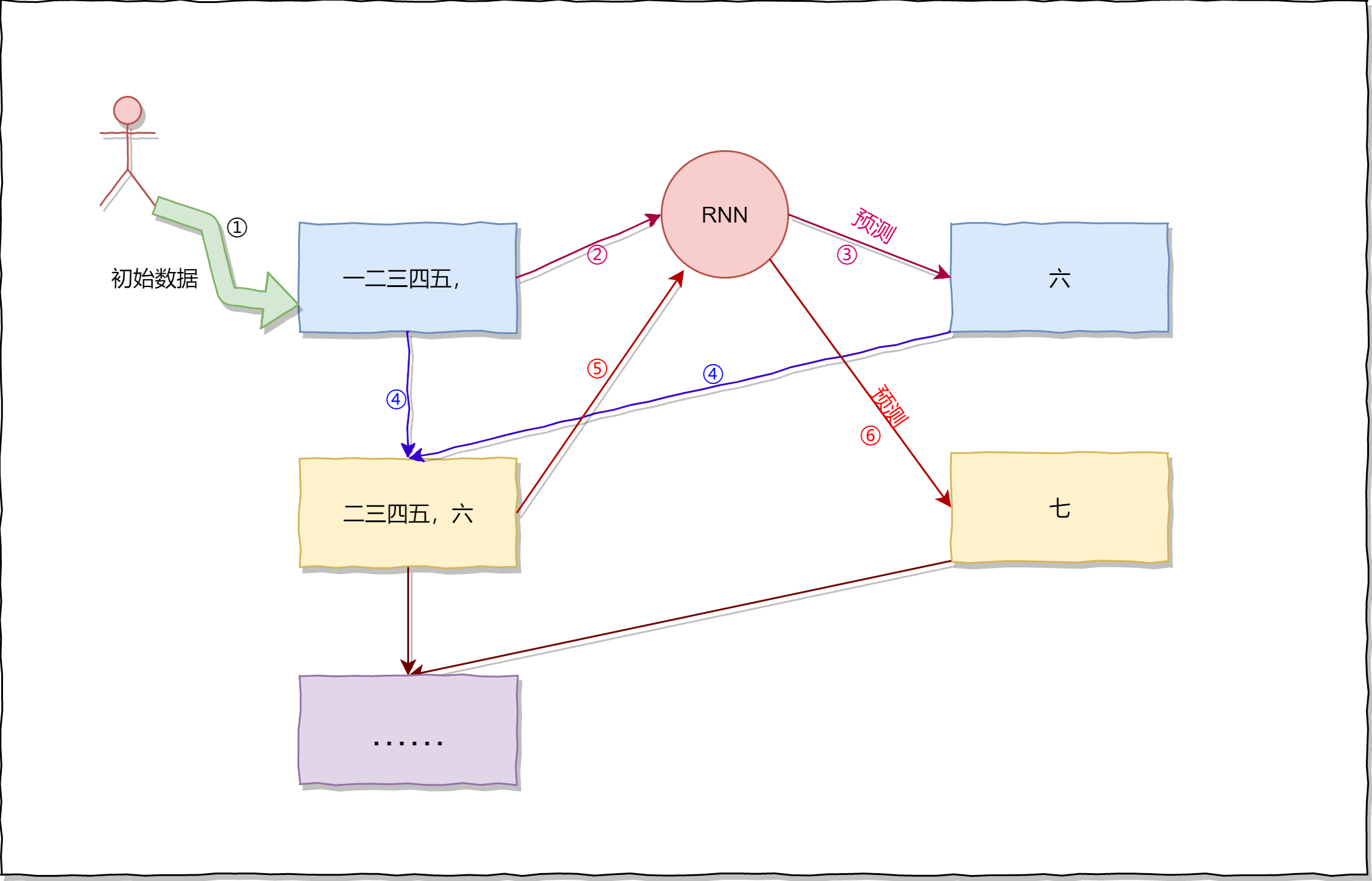## 代码实现

• DISALLOWED_WORDS：如果在诗中出现了DISALLOWED_WORDS，则舍弃这首诗。
``````# 诗data的地址
poetry_data_path = "./data/poetry.txt"
# 如果诗词中出现这些词，则将诗舍弃
DISALLOWED_WORDS = ['（', '）', '(', ')', '__', '《', '》', '【', '】', '[', ']']
# 取3000个字作诗,其中包括空格字符
WORD_NUM = 3000
# 将出现少的字使用空格代替
UNKONW_CHAR = " "
# 根据前6个字预测下一个字，比如说根据“寒随穷律变，”预测“春”
TRAIN_NUM = 6
``````

### 读取文件

• 必须是五言诗（不过下面的代码无法完全保证是五言诗），同时至少要有两句诗
• 不能出现上文中定义的DISALLOWED_WORDS

1. 获得符合要求的诗：`(len(poetry)-1) % 6`，每一首五言诗，包括“，。”一共有\(6*n\) 个字，同时每一首诗是以 “\n” 结尾的，因为我们`(len(poetry)-1)%6==0`则就代表符合要求。同时五言诗的第6个字符是”，”——> 使用`poetrys`保存。
2. 获得诗中出现的字符。——>使用`all_word`保存。
``````# 保存诗词
poetrys = []
# 保存在诗词中出现的字
all_word = []

with open(poetry_data_path,encoding="utf-8") as f:
for line in f:
# 获得诗的内容
poetry = line.split(":").replace(" ","")
flag = True
# 如果在句子中出现'（', '）', '(', ')', '__', '《', '》', '【', '】', '[', ']'则舍弃
for dis_word in DISALLOWED_WORDS:
if dis_word in poetry:
flag = False
break

# 只需要5言的诗（两句诗包括标点符号就是12个字），假如少于两句诗则舍弃
if  len(poetry) < 12 or poetry != '，' or (len(poetry)-1) % 6 != 0:
flag = False

if flag:
# 统计出现的词
for word in poetry:
all_word.append(word)
poetrys.append(poetry)
``````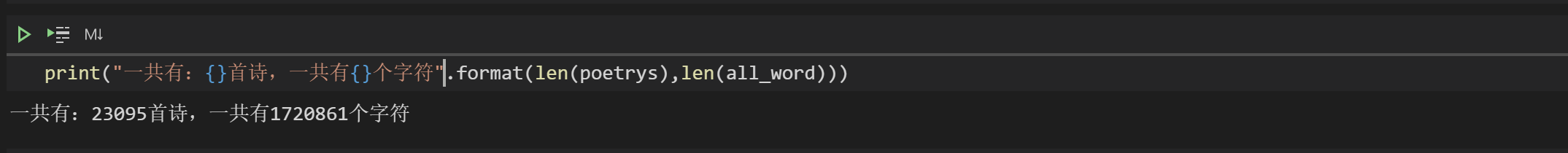### 统计字数

``````from collections import Counter
# 对字数进行统计
counter = Counter(all_word)
# 根据出现的次数，进行从大到小的排序
word_count = sorted(counter.items(),key=lambda x : -x)
most_num_word,_ = zip(*word_count)
# 取前2999个字，然后在最后加上" "
use_words = most_num_word[:WORD_NUM - 1] + (UNKONW_CHAR,)
``````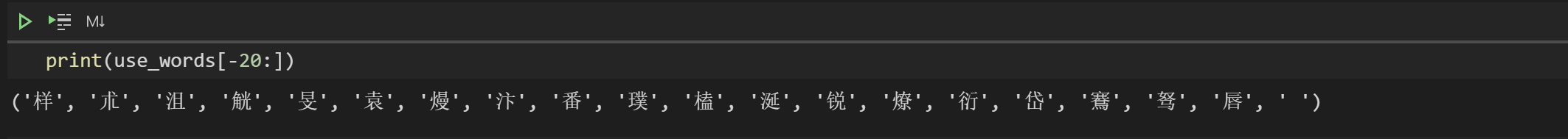### 构建word 与 id的映射

“唐” ——> 0 ；”宋”——>1；”明”——>2；进行one-hot编码后，则就变成了：

• 唐：[1,0,0]
• 宋：[0,1,0]
• 明：[0,0,1]

``````# word 到 id的映射 {'，': 0,'。': 1,'\n': 2,'不': 3,'人': 4,'山': 5,……}
word_id_dict = {word:index for index,word in enumerate(use_words)}

# id 到 word的映射 {0: '，',1: '。',2: '\n',3: '不',4: '人',5: '山',……}
id_word_dict = {index:word for index,word in enumerate(use_words)}
``````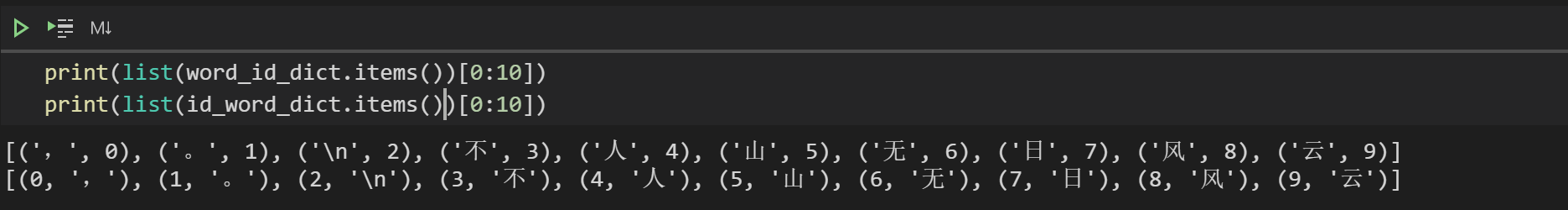### 转成one-hot代码

• word_to_one_hot将一个字转成one-hot 形式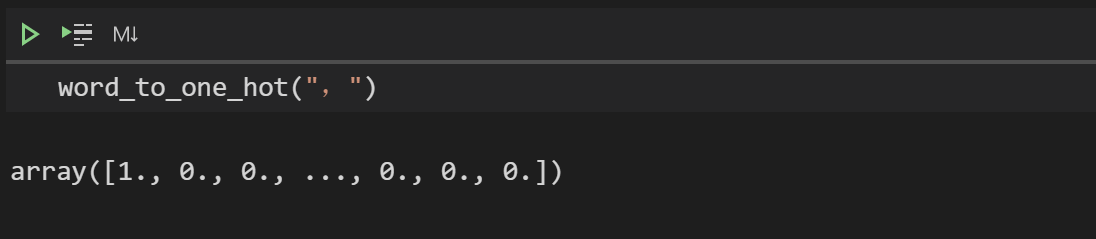• phrase_to_one_hot 将一个句子转成one-hot形式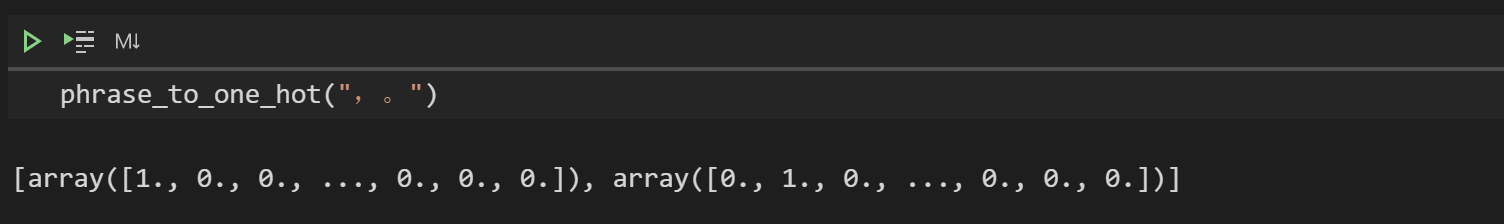``````import numpy as np
def word_to_one_hot(word):
"""将一个字转成onehot形式

:param word: [一个字]
:type word: [str]
"""
one_hot_word = np.zeros(WORD_NUM)
# 假如字是生僻字，则变成空格
if word not in word_id_dict.keys():
word = UNKONW_CHAR
index = word_id_dict[word]
one_hot_word[index] = 1
return one_hot_word

def phrase_to_one_hot(phrase):
"""将一个句子转成onehot

:param phrase: [一个句子]
:type poetry: [str]
"""
one_hot_phrase = []
for word in phrase:
one_hot_phrase.append(word_to_one_hot(word))
return one_hot_phrase
``````

### 随机打乱数据

``````np.random.shuffle(poetrys)
``````

### 构建训练集``````X_train_word = []
Y_train_word = []

for poetry in poetrys:
for i in range(len(poetry)):
X = poetry[i:i+TRAIN_NUM]
Y = poetry[i+TRAIN_NUM]
if "\n" not in X and "\n" not in Y:
X_train_word.append(X)
Y_train_word.append(Y)
else:
break
``````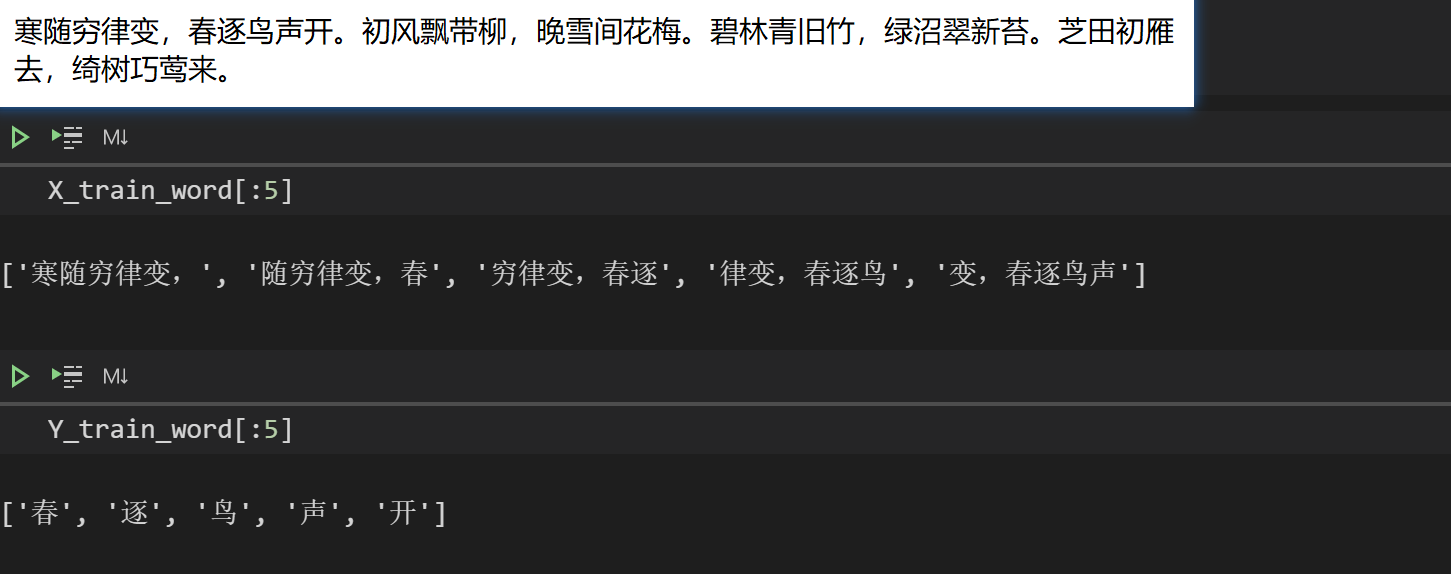### 构建模型

• keras：2.4.3：如果想使用我训练好的模型，请保持版本一致。如果自己训练的话，就无所谓了。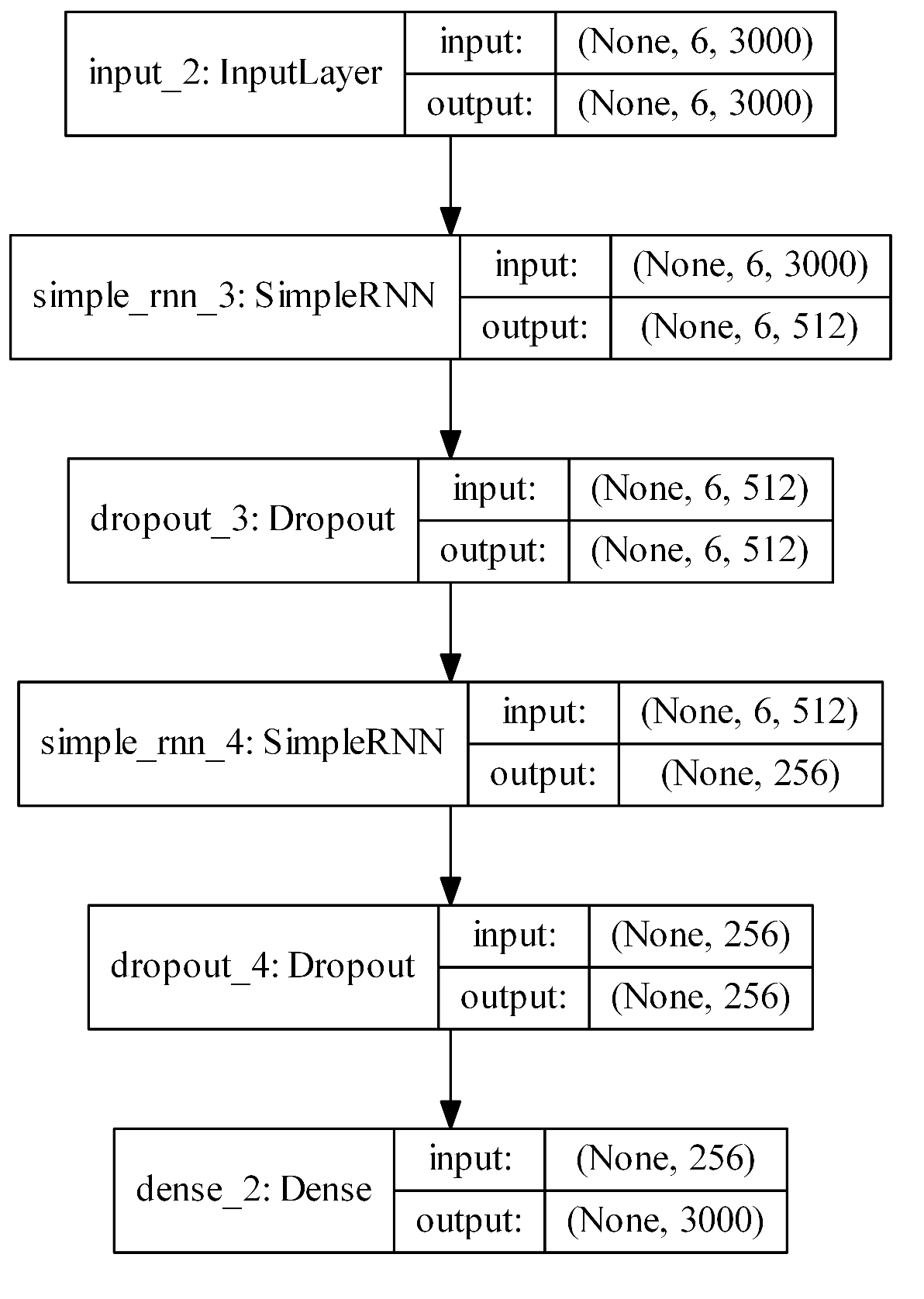``````import keras
from keras.callbacks import LambdaCallback,ModelCheckpoint
from keras.models import Input, Model
from keras.layers import  Dropout, Dense,SimpleRNN
from keras.utils import plot_model

def build_model():
print('building model')
# 输入的dimension
input_tensor = Input(shape=(TRAIN_NUM,WORD_NUM))
rnn = SimpleRNN(512,return_sequences=True)(input_tensor)
dropout = Dropout(0.6)(rnn)

rnn = SimpleRNN(256)(dropout)
dropout = Dropout(0.6)(rnn)
dense = Dense(WORD_NUM, activation='softmax')(dropout)

model = Model(inputs=input_tensor, outputs=dense)
model.compile(loss='categorical_crossentropy', optimizer=optimizer, metrics=['accuracy'])
model.summary()
# 画出模型图
# plot_model(model, to_file='model.png', show_shapes=True, expand_nested=True, dpi=500)
return  model
``````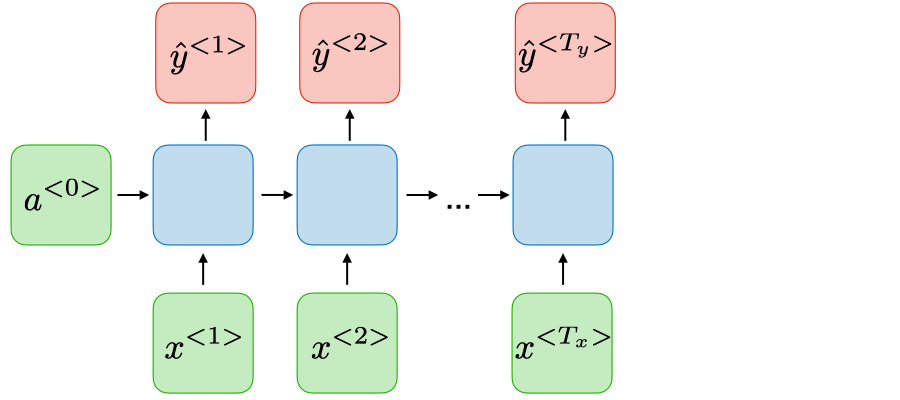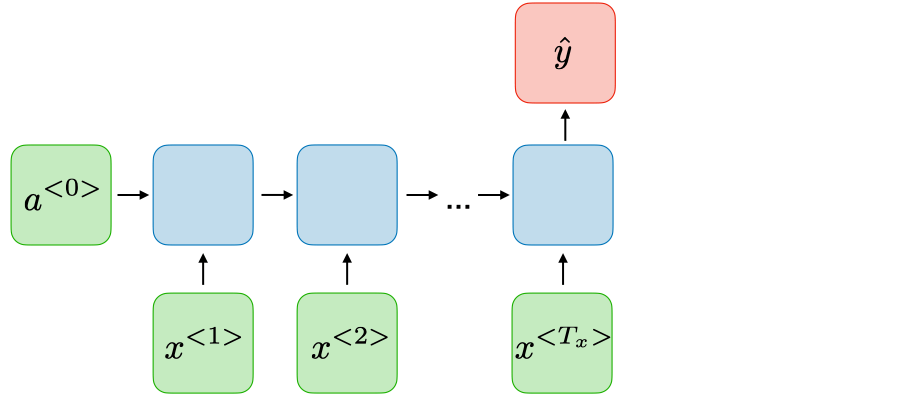``````model = build_model()
``````

### 批加载数据

so，使用keras训练的时候，不能使用fit（因为fit需要一次将数据集全部放入RAM中），而应该使用fit_generator，关于其使用推荐看看：Keras 如何使用fit和fit_generator

``````import math
def get_batch(batch_size = 32):
"""源源不断产生产生one-hot编码的训练数据

:param batch_size: [一次产生训练数据的大小], defaults to 32
:type batch_size: int, optional
:yield: [返回X（np.array(X_train_batch)）和Y（np.array(Y_train_batch)）]
:rtype: [X.shape为(batch_size, 6, 3000) , Y.shape数据的shape(batch_size, 3000)]
"""
# 确定每轮有多少个batch
steps = math.ceil(len(X_train_word) / batch_size)
while True:
for i in range(steps):
X_train_batch = []
Y_train_batch = []
X_batch_datas = X_train_word[i*batch_size:(i+1)*batch_size]
Y_batch_datas = Y_train_word[i*batch_size:(i+1)*batch_size]

for x,y in zip(X_batch_datas,Y_batch_datas):
X_train_batch.append(phrase_to_one_hot(x))
Y_train_batch.append(word_to_one_hot(y))
yield np.array(X_train_batch),np.array(Y_train_batch)
``````

### 训练的过程中生成诗句``````def predict_next(x):
""" 根据X预测下一个字符

:param x: [输入数据]
:type x: [x的shape为(1,TRAIN_NUM,WORD_NUM)]
:return: [最大概率字符的索引，有可能为为2999，也就是预测的字符可能为“ ”]
:rtype: [int]
"""
predict_y = model.predict(x)
# 获得最大概率的索引
index = np.argmax(predict_y)
return index

def generate_sample_result(epoch, logs):
"""生成五言诗

:param epoch: [目前模型训练的epoch]
:type epoch: [int]
:param logs: [模型训练日志]
:type logs: [list]
"""
# 每个epoch都产生输出
if epoch % 1 == 0:
# 根据“一朝春夏改，”生成诗
predict_sen = "一朝春夏改，"
predict_data = predict_sen
# 生成的4句五言诗（4 * 6 = 24）
while len(predict_sen) < 24:
X_data = np.array(phrase_to_one_hot(predict_data)).reshape(1,TRAIN_NUM,WORD_NUM)
# 根据6个字符预测下一个字符
y = predict_next(X_data)
predict_sen = predict_sen+ id_word_dict[y]
# “寒随穷律变，” ——> “随穷律变，春”
predict_data = predict_data[1:]+id_word_dict[y]
# 将数据写入文件
with open('out/out.txt', 'a',encoding='utf-8') as f:
f.write(write_data+'\n')
``````

### 开始训练

``````batch_size = 2048
model.fit_generator(
generator=get_batch(batch_size),
verbose=True,
steps_per_epoch=math.ceil(len(X_train_word) / batch_size),
epochs=1000000,
callbacks=[
ModelCheckpoint("poetry_model.hdf5",verbose=1,monitor='val_loss',period=1),
# 每次完成一个epoch会调用generate_sample_result产生五言诗
LambdaCallback(on_epoch_end=generate_sample_result)
]
)
``````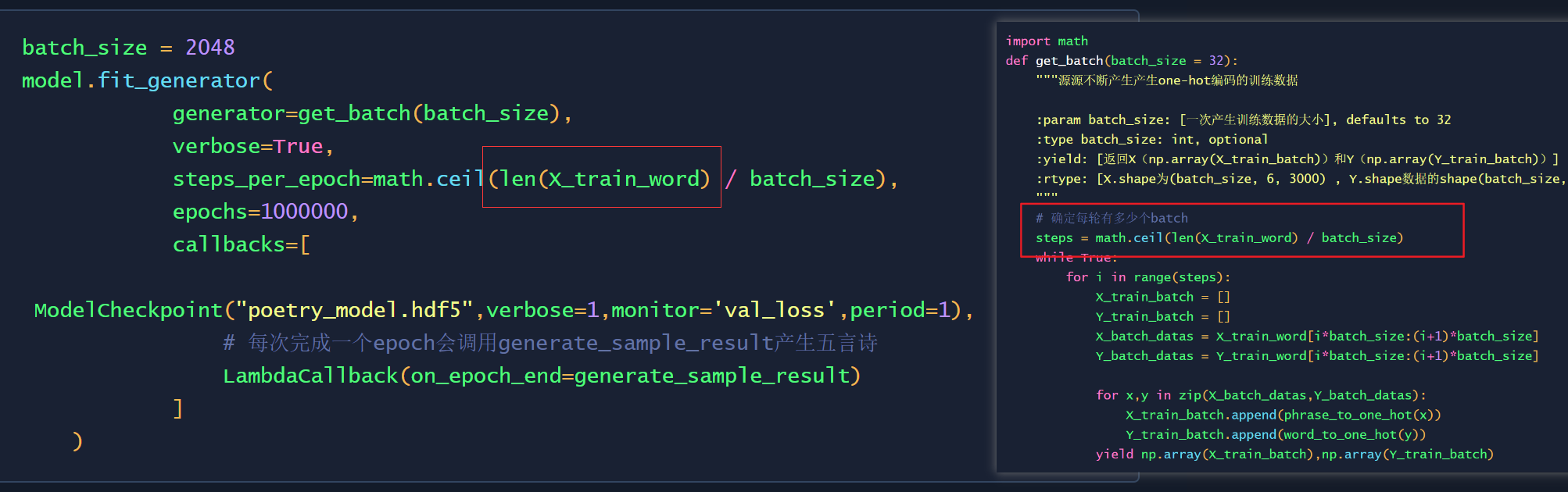## 总结

### 参考

1. RNN模型与NLP应用(6/9)：Text Generation (自动文本生成)
2. Poems_generator_Keras
3. 深度学习框架PyTorch：入门与实践
4. 用Keras实现RNN+LSTM的模型自动编写古诗
5. Keras-SimpleRNN
6. Keras-fit_generator
7. 数据挖掘入门系列教程（十一）之keras入门使用以及构建DNN网络识别MNIST
8. Keras 如何使用fit和fit_generator